# Hex Planets Dev Blog I: Generating the Hex Sphere

Hi there!

I’m Daftcube, and I’ve started a project regarding the procedural generation of convincing spherical planets partitioned into hexagons. I’m not sure how far I want to take this project, but I’ve learned a lot of useful techniques that I think are worth sharing. Plus, the pictures are pretty!

Of course, the first step to creating procedural hexagon planets are to actually, well, generate the hex spheres!

### The Trick to Spherical Hexagons: Icospheres and Dual Polyhedrons

In geometry, we can construct some shapes from others. Specifically, if we take a 3d object and replace all of its faces with vertices and create edges between them across their old common edges, we can produce what is called a geometric dual.

Why does this matter? Turns out, the geometric dual of an icosahedron is a hex sphere! Here’s a quick sketch to demonstrate this bad paint drawing!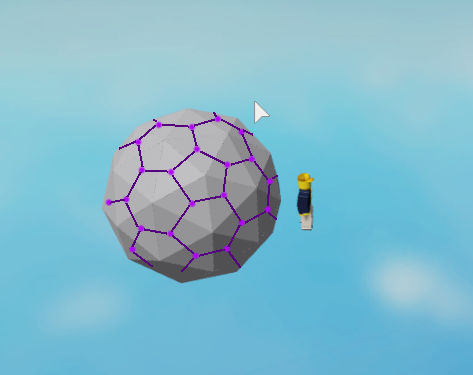The above drawing connects the vertices along the triangle’s common edges to produce the dual.

##### Addendum I: Hex Spheres are Actually Impossible

If you look closely, you will be able to spot several pentagons in the sea of hexagons. This is unavoidable; it’s actually impossible to create a spherical polyhedron using just hexagons. A (poor excuse of a) proof of this is simple: if three adjacent hexagons lie on a flat plane, there is no way to fold them. In other words, they are locked in place and cannot fold into a 3d shape without deforming one of them. However, with pentagons, it is possible to create our target polyhedron. Thus, pentagons will be a compromise we must make.

### Constructing the Icosphere

Constructing an icosphere is fairly simple. If you take three orthogonal rectangles whose sides lengths are in the golden ratio, and then connect the closest vertices together to form triangles, you get a base icosahedron…

Then, to add geometry, one can subdivide each triangular face of the base icosahedron into smaller triangles and project those points onto a unit sphere. The following image shows a subdivision of the base icosahedron’s faces before projection.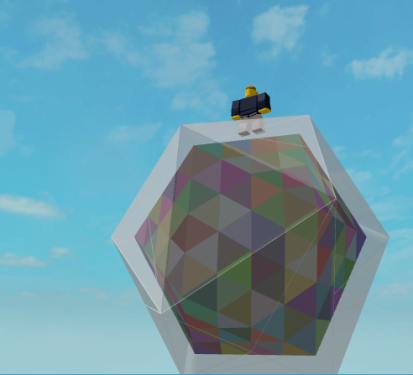…and this is a gif showing various subdivisions of an icosahedron after projection. Note that the projection is what gives the icosphere it’s spherical shape for any subdivision.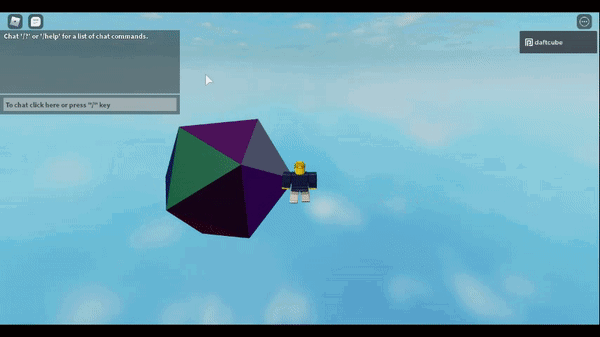One note: almost every tutorial I have seen on the internet regarding icosphere generation uses a recursive triangle partition algorithm that subdivides each triangle into four triangles in the following configuration…

This solution is valid, but has a key drawback in our use case. Because the recursive method subdivides each triangle into four triangles each iteration, our growth in geometry is exponential and gets hard to control.

But turns out, if we look at Wikipedia’s list of geodesic polyhedra, we can see that there actually exists many more valid configurations of a geodesic sphere that can be constructed from icospheres. Specifically, if you use the iterative method outlined in the above figure, you can construct any of the following icospheres and hex spheres shown in this Wikipedia table!

I built an algorithm to partition the sides of the base icosphere into this configuration, and it has given a much finer degree of control over the types of spheres that can be represented.

### Constructing the Dual of the Icosphere

Constructing the actual hex sphere from the icosphere was actually fairly trivial. Using the triangles, I made an adjacency map that connected every vertex with its neighbors. Using the points as keys, (and a modified version of Cantor’s pairing function,) I created a table that would represent that vertex’s new polygon in the dual. Finally, for each triangle face on the icosphere, I calculated its midpoint and added it to the polygon tables of its vertices.

The results look pretty awesome.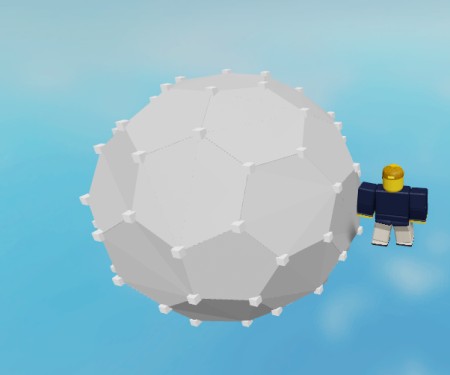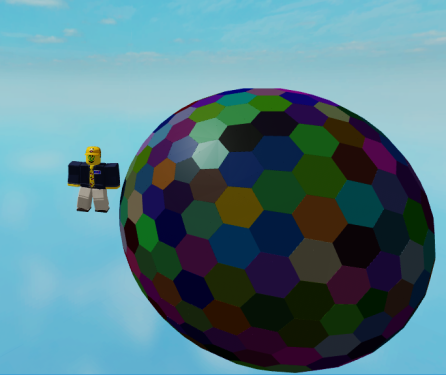There is definitely room for improvement here. The adjacency graph construction has a lot of duplicate vertex iterations. But, I figured for now it was “good enough for government work.” Besides, the spheres that will be used in the project will probably be cached before runtime anyway.

Whenever you do any math involving spheres and regular shapes, you oftentimes get distortion. This is because 2D space cannot accurately be mapped to spherical space without some distortion. You probably can recognize this distortion from maps, where Greenland is often portrayed as far larger than it really is.

My friend gave me the idea to visualize the distortion, so we wrote a quick algorithm to shade the sphere based on distortion, where distortion was defined by the ratio of the smallest side of a polygon to its longest side. More perfect polygons will have equal sizes and lie closer to one, while more distorted polygons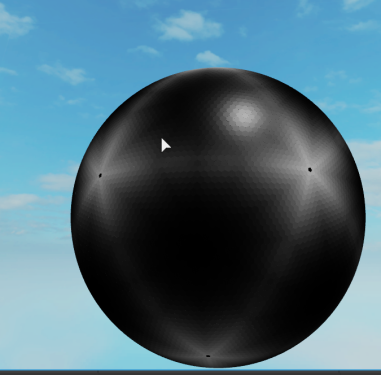Turns out, the hexagons are most distorted around the pentagons.

### Next Steps: Altitude Maps via Plate Tectonics!

Well, I got a sphere. The next logical step is to apply some sort of height data to the sphere. I think a cool way to do this would be to define tectonic plates on the surface, and then generate the heightmap based on the interactions between the edges of the plates. For more reading, see this relevant Wikipedia article.

### Acknowledgements

My good friend and Applied Mathematics major DarkInfernoDrago helped tremendously with this project so far.

42 Likes

This should go in #resources:community-tutorials

This is not a tutorial; while I outline my process for achieving the spheres, this post was not meant to be a tutorial and leaves out some critical implementation details that weren’t immediately relevant to the presentation.

If people are interested in a tutorial, I’m happy to create a more extended writeup sometime in the future!

7 Likes

It’s pretty useful for me. A new tips for buildingDamn looks amazing would you be willing to release the code for it? i want to see how this was coded.

I would really appreciate a tutorial because I know how to make triangles but I don’t know how to increase the verticies or make a Ico Sphere.

Yo this is awesome! It’s great to see someone else taking an interest in computational geometry. If anyone’s interested, I implemented and open sourced my own polyhedron generation code, which can generate icosahedrons, goldberg polyhedrons, icospheres, and more with a few specific conway operations (dual, triakis, and truncate).

5 Likes